香港萬年曆 世界電壓表 世界電視頻道表 國際電話編碼 世界貨幣代號 實用計算程式 加盟創業 裝修工程 回到主頁

USEFUL TOOLS

 面積 及 周長 體積 及 總面積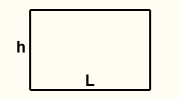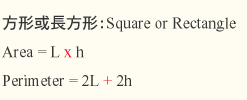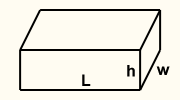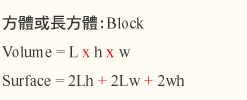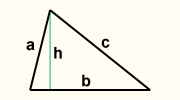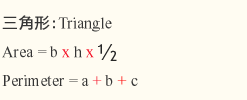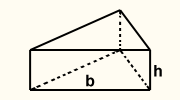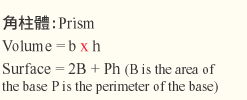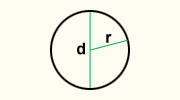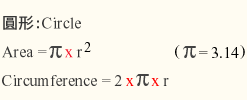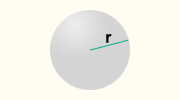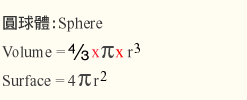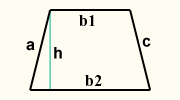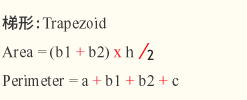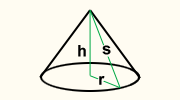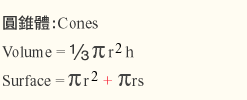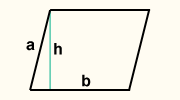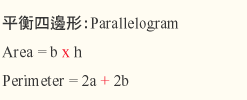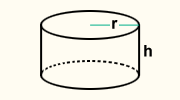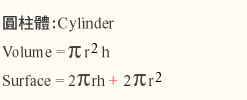UK UNITS (Length) UK UNITS (Area) UK UNITS (Volume) UK UNITS (Weight) 1 foot = 12 inches 1 sq. foot = 144 sq. inches 1 cu. foot = 1728 cu. inches 1 pound = 16 ounces 1 yard = 3 feet 1 sq. yard = 9 sq. feet 1 cu. yard = 27 cu. feet 1 stone = 14 pounds 1 mile = 5280 feet 1 acre = 4840 sq. yards 1 barrel = 58 cu. feet 1 quarter = 28 pounds 1 mile = 1760 yards 1 sq. mile = 640 acres 1 ton = 100 cubic feet 1 ton = 2240 pounds UK UNITS (Capacity) Speed Meas. Water Meas. Metric Conversion 1 pint = 4 gills 15 mph = 22 feet per sec. 1 litre water = weight 1 kg 1 inch = 2.54cm 1 quart = 2 pints 1 knot = 1 nautical mph 1 cu. metre = weight 1 ton 1 foot = 30.48cm 1 gallon = 4 quarts 1 miles = 1.61 km 1 gallon = 10.22 lb 1 yard = 0.9144m 1 bulk barrel = 36 gallons 1 gallon = 4.54 litres 1 kg = 2.20 pounds輸入您的搜尋字詞 提交搜尋表單

 友情鏈結：開自己的餐廳  |  品酒會  |  婚宴葡萄酒 | 葡萄酒入貨網 | 網上買酒 | 志豐集團 | 廚房工程 | 清拆工程 | | 品牌設計 | 成為 KOL 美食博客 | 網上買酒 40% OFF | 辦公室裝修工程 | 開飲品店網 Copyright (C) Est. since 2003 Newsgroup Directory 香港討論區目錄 All right reserved 免責聲明